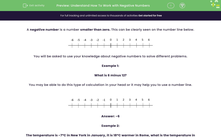# Understand How To Work with Negative Numbers

In this worksheet, students will use their knowledge and understanding of negative numbers to solve a variety of calculations and worded problems.Key stage:  KS 2

Curriculum topic:   Number: Number and Place Value

Curriculum subtopic:   Use Negative Numbers

Difficulty level:#### Worksheet Overview

negative number is a number smaller than zero. This can be clearly seen on the number line below.Example 1:

What is 6 minus 12?

You may be able to do this type of calculation in your head or it may help you to use a number line.Example 2:

The temperature is -7°C in New York in January, it is 18°C warmer in Rome, what is the temperature in Rome?

Count on from -7°C to 0 and then add on another 11 so in total, you have added 18